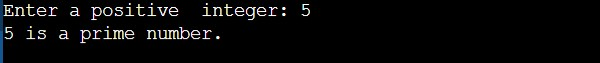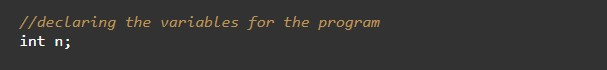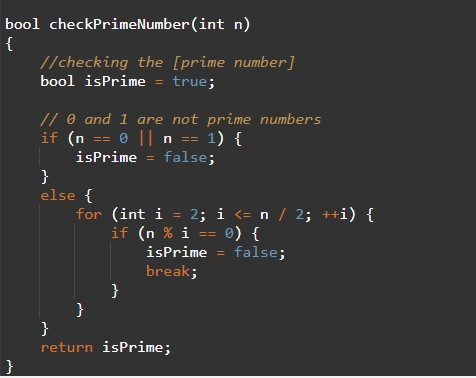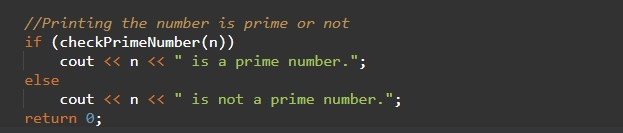# C++ Program to Check Whether a Number is Prime or Not

In this tutorial you will learn about the C++ Program to Check Whether a Number is Prime or Not and its application with practical example.

## C++ Program to Check Whether a Number is Prime or Not

In this tutorial, we will learn to create a C++ program that will Check Whether a Number is Prime or Not using C++ programming.

## Prerequisites

Before starting with this tutorial we assume that you are best aware of the following C++ programming topics:

• Operators in C++ Programming.
• Basic Input and Output function in C++ Programming.
• Basic C++ programming.
• For loop in C++ programming.
• Conditional Statements in C++ programming.
• Arithmetic operations in C++ Programming.

## Program to Check Whether a Number is Prime or Not:-

In c++ programming, it is possible to take numerical input from the user and check if that number is Prime Number or not. The prime number means a number that is only divisible by itself.

With the help of this program, we can Check Whether a Number is Prime or Not.

## Output:-In the above program, we have first initialized the required variable.• no= it will hold the integer value of the input.
• i = it will hold the integer value.
• flag = it will hold the integer value.

Input message for the user for the integer value.Program Logic Code.Printing output prime number or not.In this tutorial we have learn about the C++ Program to Check Whether a Number is Prime or Not and its application with practical example. I hope you will like this tutorial.